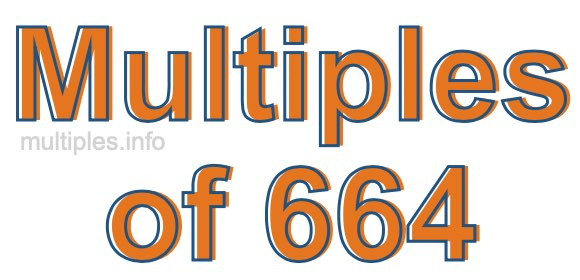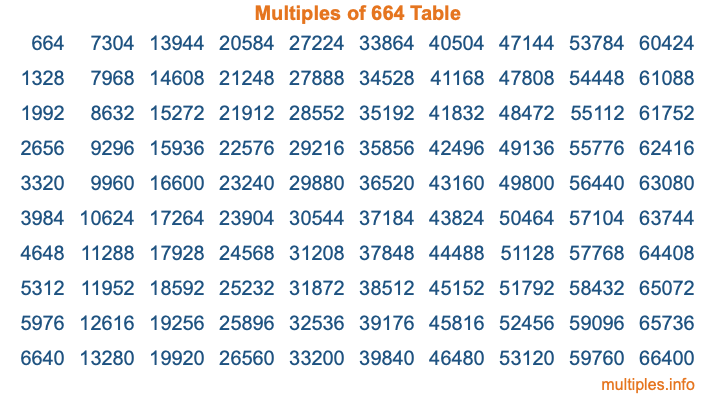Multiples of 664Welcome to the Multiples of 664 page. Here we will first teach you everything you will ever need to know about the multiples of 664, and then give you a study guide summary of everything we taught you to make sure you remember it all. Use this page to look up facts and learn information about the multiples of 664. This page will make you a multiples of six hundred sixty-four expert!

Definition of Multiples of 664
Multiples of 664 are all the numbers that when divided by 664 equal an integer. Each of the multiples of 664 are called a multiple. A multiple of 664 is created by multiplying 664 by an integer.

Therefore, to create a list of multiples of 664, you start with 1 multiplied by 664, then 2 multiplied by 664, then 3 multiplied by 664, and so on for as long as you want. Thus, the list of the first five multiples of 664 is 664, 1328, 1992, 2656, and 3320. To see a larger list of multiples of 664, see the printable image of Multiples of 664 further down on this page. We also have a category where you can choose any nth multiple of 664.

Multiples of 664 Checker
The Multiples of 664 Checker below checks to see if any number of your choice is a multiple of 664. In other words, it checks to see if there is any number (integer) that when multiplied by 664 will equal your number. To do that, we divide your number by 664. If the the quotient is an integer, then your number is a multiple of 664.

Is  a multiple of 664?

Least Common Multiple of 664 and ...
A Least Common Multiple (LCM) is the lowest multiple that two or more numbers have in common. This is also called the smallest common multiple or lowest common multiple and is useful to know when you are adding our subtracting fractions. Enter one or more numbers below (664 is already entered) to find the LCM.

Check out our LCM Calculator if you need more details about the Least Common Multiple or if you need the LCM for different numbers for adding and subtraction fractions.

nth Multiple of 664
As we stated above, 664 is the first multiple of 664, 1328 is the second multiple of 664, 1992 is the third multiple of 664, and so on. Enter a number below to find the nth multiple of 664.

th multiple of 664

Multiples of 664 vs Factors of 664
664 is a multiple of 664 and a factor of 664, but that is where the similarities end. All postive multiples of 664 are 664 or greater than 664. All positive factors of 664 are 664 or less than 664.

Below is the beginning list of multiples of 664 and the factors of 664 so you can compare:

Multiples of 664: 664, 1328, 1992, 2656, 3320, etc.

Factors of 664: 1, 2, 4, 8, 83, 166, 332, 664

As you can see, the multiples of 664 are all the numbers that you can divide by 664 to get a whole number. The factors of 664, on the other hand, are all the whole numbers that you can multiply by another whole number to get 664.

It's also interesting to note that if a number (x) is a factor of 664, then 664 will also be a multiple of that number (x).

Multiples of 664 vs Divisors of 664
The divisors of 664 are all the integers that 664 can be divided by evenly. Below is a list of the divisors of 664.

Divisors of 664: 1, 2, 4, 8, 83, 166, 332, 664

The interesting thing to note here is that if you take any multiple of 664 and divide it by a divisor of 664, you will see that the quotient is an integer.

Multiples of 664 Table
Below is an image of the first 100 multiples of 664 in a table. The table is in chronological order, column by column. The first column has the first ten multiples of 664, the second column has the next ten multiples of 664, and so on.The Multiples of 664 Table is also referred to as the 664 Times Table or Times Table of 664. You are welcome to print out our table for your studies.

Negative Multiples of 664
Although not often discussed or needed in math, it is worth mentioning that you can make a list of negative multiples of 664 by multiplying 664 by -1, then by -2, then by -3, and so on, to get the following list of negative multiples of 664:

-664, -1328, -1992, -2656, -3320, etc.

Multiples of 664 Summary
Below is a summary of important Multiples of 664 facts that we have discussed on this page. To retain the knowledge on this page, we recommend that you read through the summary and explain to yourself or a study partner why they hold true.

There are an infinite number of multiples of 664.

A multiple of 664 divided by 664 will equal a whole number.

664 divided by a factor of 664 equals a divisor of 664.

The nth multiple of 664 is n times 664.

The largest factor of 664 is equal to the first positive multiple of 664.

664 is a multiple of every factor of 664.

664 is a multiple of 664.

A multiple of 664 divided by a divisor of 664 equals an integer.

664 divided by a divisor of 664 equals a factor of 664.

Any integer times 664 will equal a multiple of 664.

Multiples of a Number
Here you can get the multiples of another number, all with the same attention to detail as we did for multiples of 664 on this page.

Multiples of
Multiples of 665
Did you find our page about multiples of six hundred sixty-four educational? Do you want more knowledge? Check out the multiples of the next number on our list!